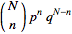# Probability and Poisson Random Variable

## Homework Statement

A trial consists of throwing two dice. The result is counted as successful if the sum of
the outcomes is 12. What is the probability that the number of successes in 36 such trials
is greater than one? What is this probability if we approximate its value using the Poisson
random variable?

## The Attempt at a Solution

So there is a 1/36 chance that throwing two dice results in a sum of 12. I'm not sure where to go from here though.

The trials are independent since you are doing each trial separately. What do you know about the probability of independent events?

P(EF) = P(E)P(F), so i guess i'd say E represents the event that the sum of the dice is 12 and F is the event that more than one trial is a success?

Ray Vickson
Homework Helper
Dearly Missed

## Homework Statement

A trial consists of throwing two dice. The result is counted as successful if the sum of
the outcomes is 12. What is the probability that the number of successes in 36 such trials
is greater than one? What is this probability if we approximate its value using the Poisson
random variable?

## The Attempt at a Solution

So there is a 1/36 chance that throwing two dice results in a sum of 12. I'm not sure where to go from here though.

Until you understand the basics, you should work with a simpler case.

So, to start: suppose you do two independent trials. What is the probability that neither trial gives a '12'? What is the probability that both trials give a '12'? What is the probability that exactly one of the two trials gives a '12'?

Now think about what would happen if you had three independent trials, then four independent trials, etc.

Bleh so apparently i need to be using a binomial distribution on this problem.So i have

N = number of trials
p = probability of a success in a given trial
n = number of expected successful trials
q = 1 - p

N = 36,
p = 1/36,
1 = 35/36,
n >= 1

The problem is that i need an actual value for n, and n could be any number larger than 1 so it's throwing me off. How would i calculate this?

Ray Vickson
Homework Helper
Dearly Missed
Bleh so apparently i need to be using a binomial distribution on this problem.So i have

N = number of trials
p = probability of a success in a given trial
n = number of expected successful trials
q = 1 - p

N = 36,
p = 1/36,
1 = 35/36,
n >= 1

The problem is that i need an actual value for n, and n could be any number larger than 1 so it's throwing me off. How would i calculate this?

The question asked for n > 1, not n >= 1. It makes a big difference. Anyway, you want the probability of the event {n ≥ 2} = {n = 2 or n = 3 or n = 4 or .... or n = 36}.

By my calculations, n = 1 - [q^N] - [N*p*q^(N-1)]

which evaluates to be 0.2642.